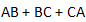# The equivalent of the block diagram in Fig-1 is given as

1.

2.

3.

4.

4Explanation :
No Explanation available for this question

# The transfer function C(s)/R(s) of the system shown is

1.   0

2.  1/s+1

3.  1/s+2

4.   2/s+3

4

1/s+1

Explanation :
No Explanation available for this question

# Output Y of the circuit shown in the figure

1.  (A+B)C+DE

2.  AB+C(D+E)

3.  (A+B)C+D+E

4.  (AB+C).DE

4

(A+B)C+DE

Explanation :
No Explanation available for this question

# The figure given below shows the circuit of which one of the following

1.  Bi-stable multi – vibrator

2.  Schmitt trigger

3.  Mono-stable multi-vibrator

4.  Astable multi-vibrator

4

Mono-stable multi-vibrator

Explanation :
No Explanation available for this question

# A full-adder can be implemented with half-adders and OR gates. A 4-bit parallel full adder without any initial carry requires

1.   8 half – adders, 4-OR gates

2.   8 half – adders, 4-OR gates

3.  7 half – adders, 4-OR gates

4.  7 half- adders, 3-OR gates

4

Explanation :
No Explanation available for this question

# A four – variable switching function has minterms m6 and m9. If literals in these minterms are complemented, then corresponding minterm numbers are

1.  m3 and m0

2.  m9 and m6

3.  m2 and m0

4.  m6 and m9

4

m3 and m0

Explanation :
No Explanation available for this question

# For the gate network shown in the figure below, which of the following gate is redundant

1.  Gate No-1

2.  Gate No-2

3.  Gate No-3

4.  Gate No-4

4

Gate No-2

Explanation :
No Explanation available for this question

# In the logic circuit shown in the figure, output X is

1.

2.

3.

4.

4Explanation :
No Explanation available for this question

# Consider the following expressions

1.   Y = f(A, B, C, D) = Σ(1, 2, 4, 7, 8 ,11, 13, 14)

2.  Y = f(A, B, C, D) = Σ(3, 5, 7, 10, 11, 12, 13, 14)

3.  Y = f(A, B, C, D) = π(0, 3, 5, 6, 9, 10, 12, 15)

4.  Y = f(A, B, C, D) = π(0, 1, 2, 4, 5, 8, 9, 15)

4

Y = f(A, B, C, D) = π(0, 1, 2, 4, 5, 8, 9, 15)

Explanation :
No Explanation available for this question

1.  F = A+B

2.  F = AB

3.  F =  A -B

3